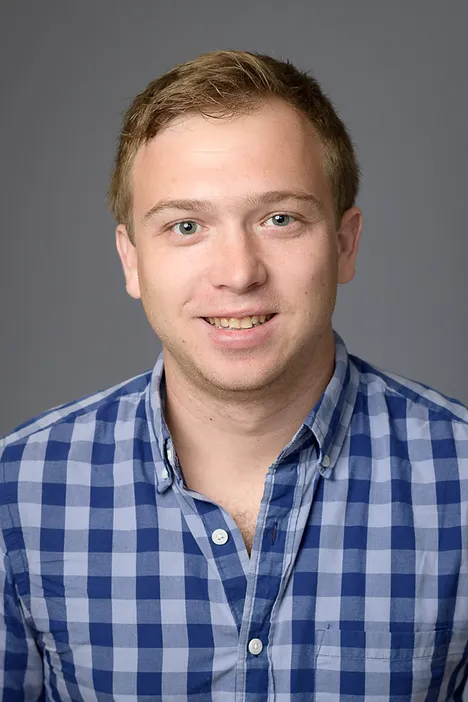In 2011, I received by B.S. in Mathematics and Physics at the University of California, San Diego and in 2017, I received my PhD in Mathematics from Purdue University, under the supervision of David Ben McReynolds.

Papers

1. Locally Equivalent Correspondences (with B. Linowitz and D. B. McReynolds). Ann. Inst. Fourier (Grenoble) 67 (2017), no. 2, 451–482.
2. Appendix to Quasi-isometric embeddings of non-uniform lattices by D. Fisher and T. Nguyen (with S. Garibaldi, D. B. McReynolds, and D. Witte Morris). Comment. Math. Helv. 95 (2020), no. 1, 37–78.
3. Effective virtual and residual properties of some arithmetic hyperbolic 3-manifolds (with J. Deblois and P. Patel). Trans. Amer. Math. Soc. 373 (2020), no. 11, 8219-8257.
4. Finiteness of maximal geodesic submanifolds in hyperbolic hybrids (with D. Fisher, J.-F. Lafont, and M. Stover). J. Eur. Math. Soc. (JEMS) 23 (2021), no. 11, 3591–3623.
5. Areas of totally geodesic surfaces of hyperbolic 3-orbifolds (with B. Linowitz and D. B. McReynolds). Pure Appl. Math. Q. 17 (2021), no. 1, 1–25.
6. Arithmeticity, superrigidity, and totally geodesic submanifolds (with U. Bader, D. Fisher, and M. Stover). Ann. of Math. (2) 193 (2021), no. 3, 837–861.
7. Arithmeticity, superrigidity, and totally geodesic submanifolds of complex hyperbolic manifolds (with U. Bader, D. Fisher, and M. Stover). Invent. Math. 233 (2023), no. 1, 169–222.
8. Arithmetic progressions in the primitive length spectrum. Available at arXiv:1602.01869 [math.GT].
9. On the genus of congruence surfaces from maximal orders (with E. Albers). Available at arXiv: 1901.07934 [math.GT].
10. Infinite-type loxodromic isometries of the relative arc graph (with C. Abbott and P. Patel). Available at: arXiv: 2109.06106 [math.GT].
11. Unmarked simple length spectral rigidity for covers (with T. Aougab, M. Lahn, and M. Loving). Available at: arXiv: 2210.16706 [math.GT].
12. A note on an effective characterization of covers with an application to higher rank representations (with T. Aougab, M. Lahn, and M. Loving). Available at: arXiv:2307.09643 [math.GT].
13. Shift maps are not type-preserving (with C. Abbott and P. Patel). Available at: arXiv: 2212.09156 [math.GT].
14. Azumaya algebras and once punctured torus bundles. Available at arXiv:2303.16309 [math.GT].

Current Teaching

M4653, Introduction to Differential Geometry I, Fall 2023

Past Teaching

University of Oklahoma:
M2423, Calculus & Analytic Geometry II, Spring 2023
M2443, Calculus & Analytic Geometry IV, Fall 2022

UC Berkeley:
M185, Complex Analysis, Fall 2022
M185, Complex Analysis, Spring 2021
M199, Directed Reading Course (Measure theory), Spring 2020
M104, Real Analysis, Spring 2020
M185, Complex Analysis, Fall 2019
M104, Real Analysis, Fall 2019

Indiana University:
M391 Introduction to Mathematical Reasoning, Spring 2019
M211 Calculus I, Fall 2018
M211 Calculus I, Spring 2017
M118 Finite Mathematics, Fall 2017

Purdue University:
MA16010 Applied Calculus I, Fall 2015
MA26600 Ordinary Differential Equations, Summer 2014
MA22100 Calculus for Technology I, Spring 2014
MA22300 Introductory Analysis I, Fall 2013
MA26200 TA for Linear Algebra And Differential Equations, Spring 2013
MA16500 TA for Analytic Geometry And Calculus II, Fall 2012
MA16200 TA for Plane Analytic Geometry And Calculus II, Spring 2012
MA26100 TA for Multivariate Calculus, Fall 2011

Nicholas MillerAssistant Professor
Department of Mathematics
University of Oklahoma

Research Interests: Hyperbolic geometry, low dimensional topology, homogeneous dynamics, and arithmetic groups.

Office: PHSC 801
Email: nickmbmiller@ou.edu

CV can be found here.